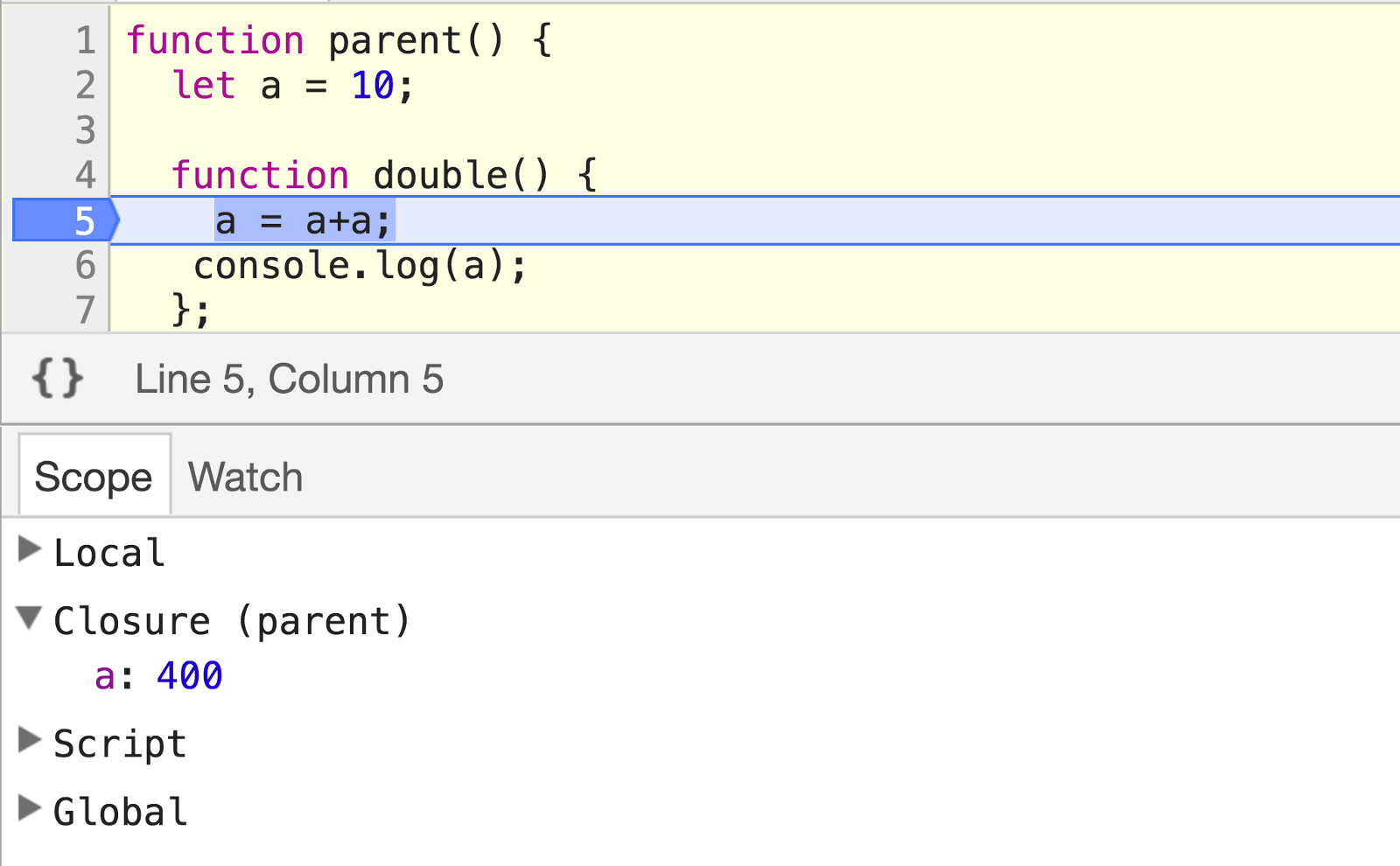# 【转】什么是JavaScript闭包？

JavaScript闭包就如同汽车的功能——不同的位置都有对应那辆车的不同组件。

JavaScript中的每一个函数都构成一个闭包，这也是JavaScript最酷的特点之一。因为没有闭包的话，实现像回调函数或者事件句柄这样的公共结构就会很困难。

``````function accelerate(force) {
// Is the car started?
// Do we have fuel?
// Are we in traction control mode?
// Many other checks...
// If all good, burn more fuel depending on
// the force variable (how hard we’re pressing the gas pedal)
}
``````### 1 — 按引用分配函数

``````function sayHello() {
console.log("hello");
};

var func = sayHello;
``````

``````func.answer = 42;

console.log(sayHello.answer); // prints 42
``````

``````func() // prints "hello"
``````

### 2 — 作用域有生命周期

``````function printA() {
var answer = 1;
};
``````
``````printA(); // this creates a scope which gets discarded right after
``````
``````printA(); // this creates a new different scope which also gets discarded right after;
``````

### 3 — 闭包跨越多个作用域

#### 当你定义一个函数，也就创建了一个闭包

``````var G = 'G';

// Define a function and create a closure
function functionA() {
var A = 'A'

// Define a function and create a closure
function functionB() {
var B = 'B'
console.log(A, B, G);
}

functionB();  // prints A, B, G

// functionB closure does not get discarded
A = 42;
functionB();  // prints 42, B, G
}

functionA();
``````

#### 不要将闭包和作用域混淆

``````// scope: global
var a = 1;
void function one() {
// scope: one
// closure: [one, global]
var b = 2;

void function two() {
// scope: two
// closure: [two, one, global]
var c = 3;

void function three() {
// scope: three
// closure: [three, two, one, global]
var d = 4;
console.log(a + b + c + d); // prints 10
}();
}();
}();
``````

``````var v = 1;

var f1 = function () {
console.log(v);
}

var f2 = function() {
var v = 2;
f1(); // Will this print 1 or 2?
};

f2();
``````

``````var f1 = function () {
console.log(v);
}

var f2 = function() {
var v = 2;
f1(); // ReferenceError: v is not defined
};

f2();
``````

### 4 — 闭包有读和写的权限

``````function outer() {
let a = 42;

function inner() {
a = 43;
}

inner();
console.log(a);
}

outer();
``````

### 5 — 闭包可以分享作用域

``````function parent() {
let a = 10;

function double() {
a = a+a;
console.log(a);
};

function square() {
a = a*a;
console.log(a);
}

return { double, square }
}

let { double, square } = parent();

double(); // prints 20
square(); // prints 400
double(); // prints 800
``````

### 最后一个测试

``````let a = 1;

const function1 = function() {
console.log(a);
a = 2
}

a = 3;

const function2 = function() {
console.log(a);
}

function1();
function2();
``````